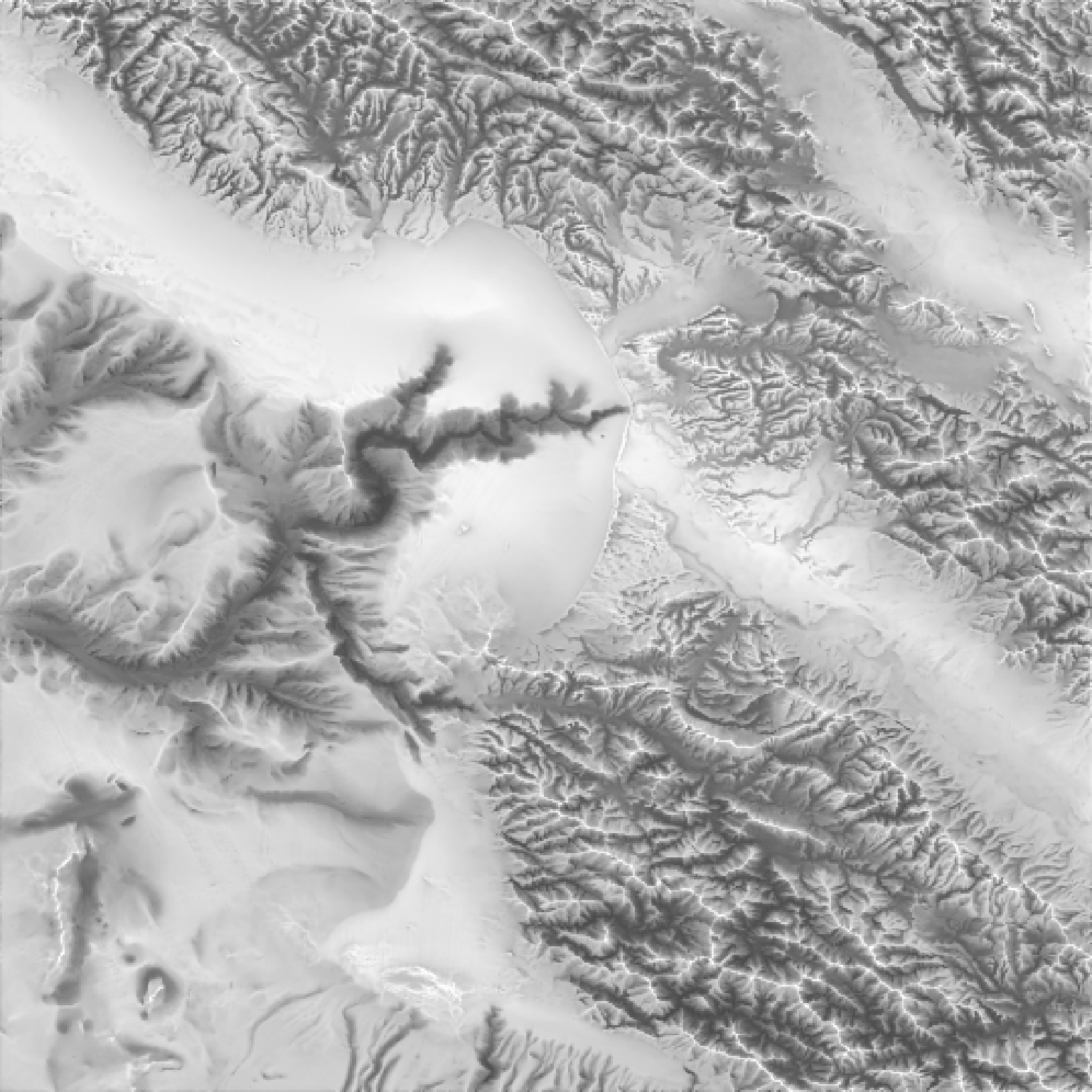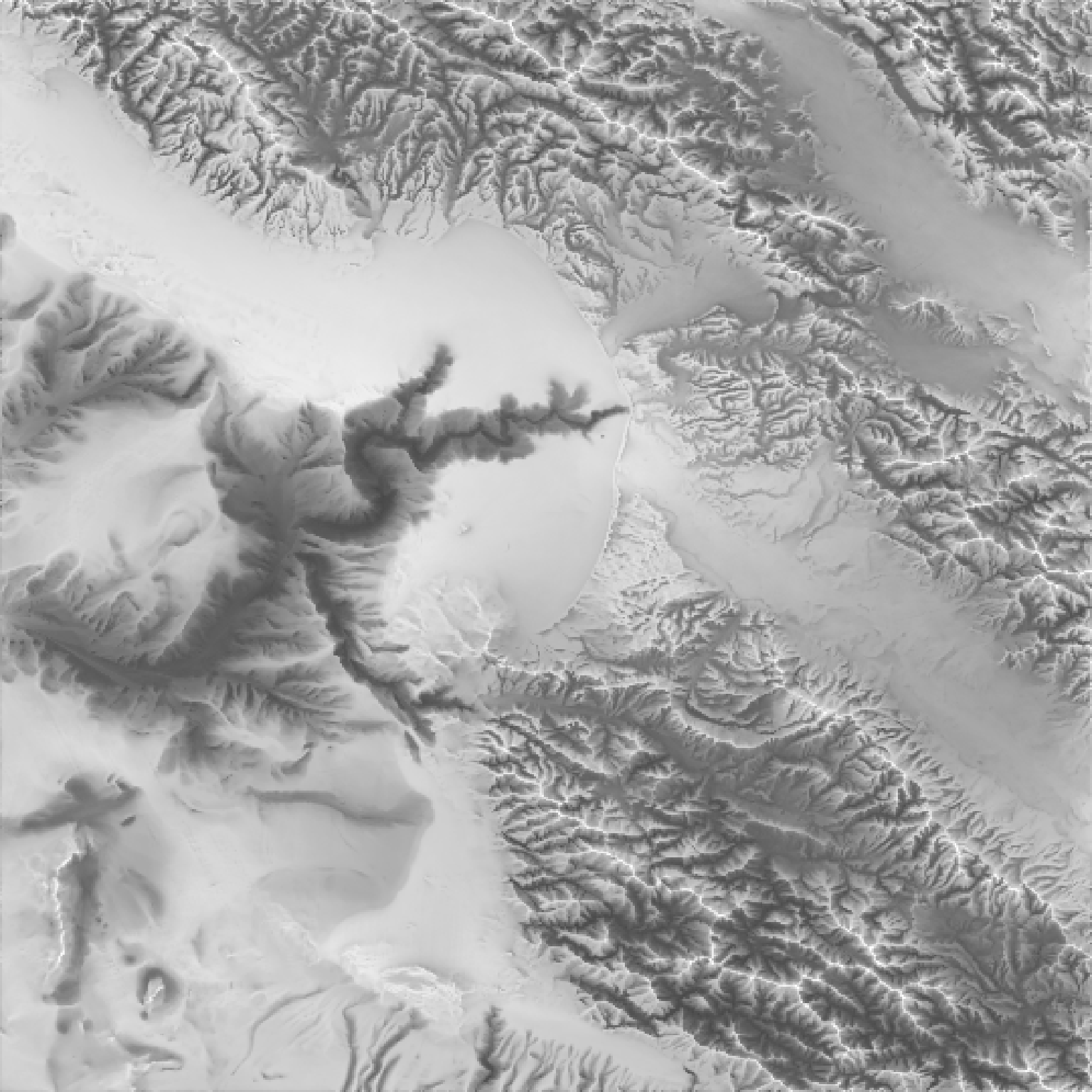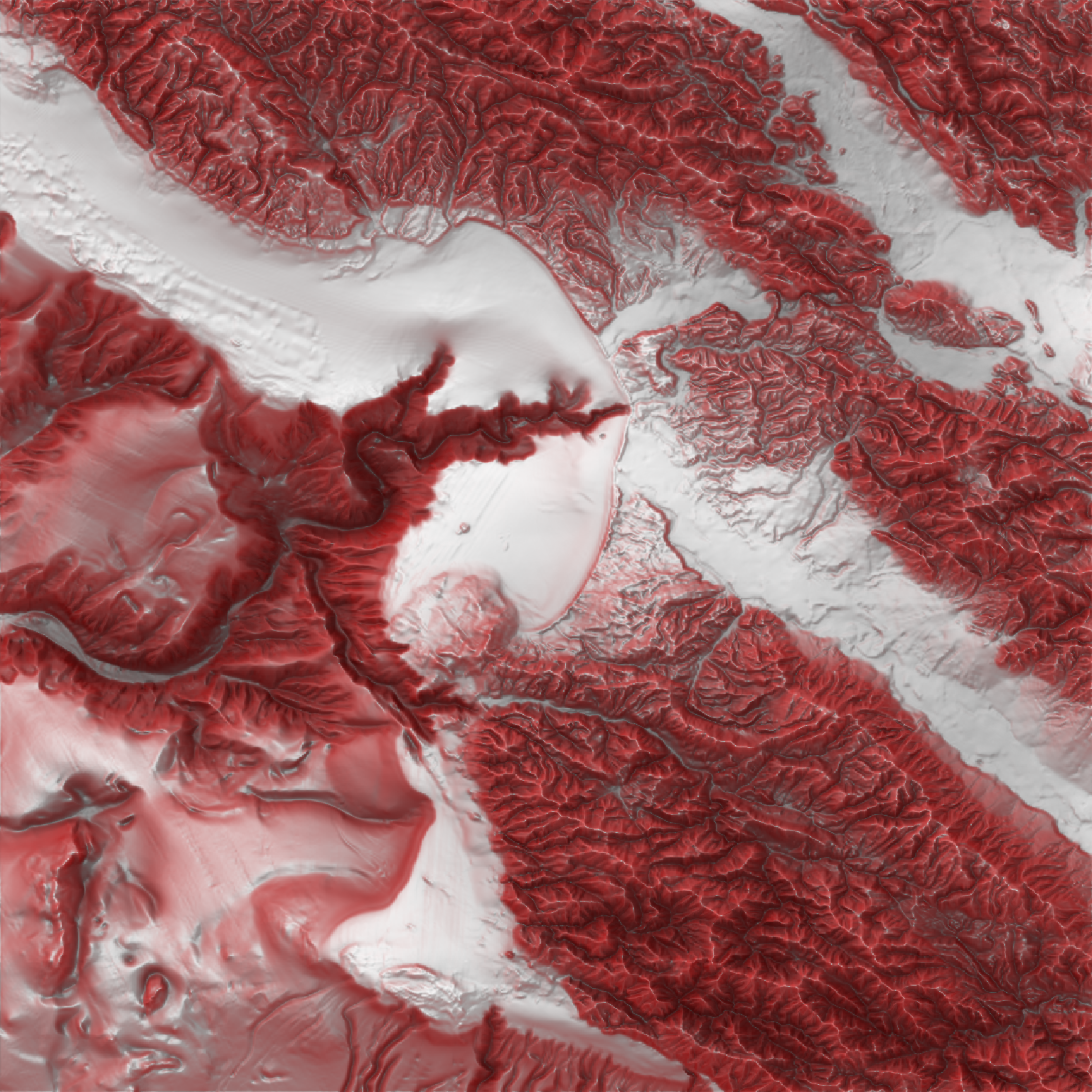ambient_shade(
heightmap,
anglebreaks = 90 * cospi(seq(5, 85, by = 5)/180),
sunbreaks = 24,
maxsearch = 30,
multicore = FALSE,
zscale = 1,
progbar = interactive(),
...
)

## Arguments

heightmap

A two-dimensional matrix, where each entry in the matrix is the elevation at that point. All points are assumed to be evenly spaced.

anglebreaks

Default 90*cospi(seq(5, 85,by =5)/180). The angle(s), in degrees, as measured from the horizon from which the light originates.

sunbreaks

Default 24. Number of rays to be sent out in a circle, evenly spaced, around the point being tested.

maxsearch

Default 30. The maximum horizontal distance that the system should propogate rays to check for surface intersections.

multicore

Default FALSE. If TRUE, multiple cores will be used to compute the shadow matrix. By default, this uses all cores available, unless the user has set options("cores") in which the multicore option will only use that many cores.

zscale

Default 1. The ratio between the x and y spacing (which are assumed to be equal) and the z axis.

Default NULL. A matrix of 1 and 0s, indicating which points on which the raytracer will operate.

Default NULL. The shadow matrix to be updated at the points defined by the argument cache_mask.

progbar

Default TRUE if interactive, FALSE otherwise. If FALSE, turns off progress bar.

...

Additional arguments to pass to the makeCluster function when multicore=TRUE.

## Examples

#Here we produce a ambient occlusion map of the montereybay elevation map.
# \dontrun{# }

#We can increase the distance to look for surface intersections maxsearch
#and the density of rays sent out around the point sunbreaks.
# \dontrun{
plot_map(ambient_shade(montereybay, sunbreaks = 24,maxsearch = 100, multicore=TRUE))# }
#Create the Red Relief Image Map (RRIM) technique using a custom texture and ambient_shade(),